# AP Physics C Electricity : Interpreting Circuit Diagrams

## Example Questions

### Example Question #1 : Interpreting Circuit Diagrams

Three resistors R1, R2, and R3 and the capacitor, C, are connected to an ideal battery V to complete the circuit as shown.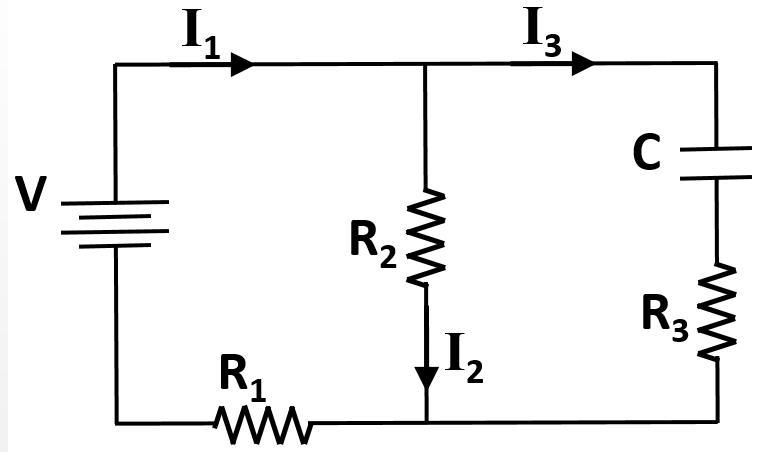After the circuit has been connected for a very long time, the currents in each branch of the circuit are measured to be some values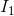,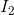, and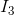, and the charge on the capacitor some value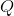.

Which of the following equations is a correct expression regarding the voltage of the circuit after a long time?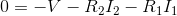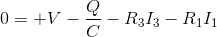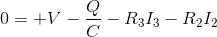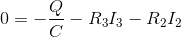Explanation:

Identifying the sum of the voltage drops and rises (Kirchoff's Loop Law) around the three possible loops of this circuit is the key to answering this question correctly. The following signs can be assigned to each of the circuit elements based on the direction of the currents given.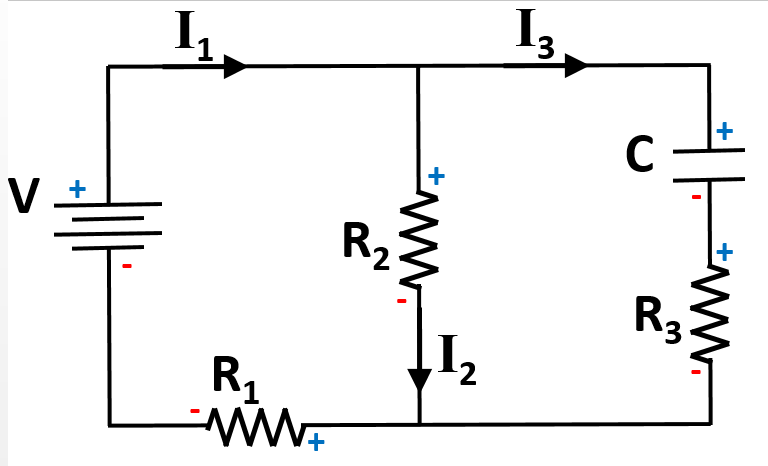Use Ohm's law and the equation for capacitance to derive terms for the voltage across each element of the circuit.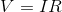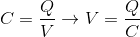There are three possible paths through the circuit, resulting in three correct equations that could be derived: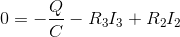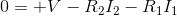Only one of the given answer options matches up correctly to these.

### All AP Physics C Electricity Resources